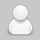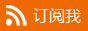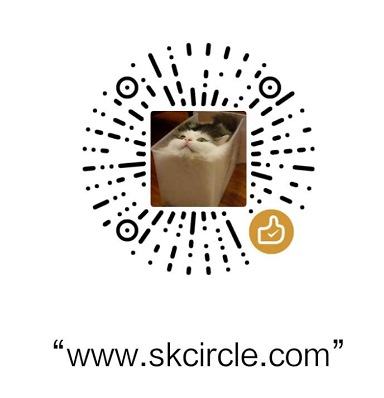#少有人走的路

## kNN算法及实例(一)

kNN算法又称为k近邻分类(k-nearest neighbor classification)算法。简单的分类就是待分类的数据与哪条已分类的数据相同，那么它们就属于同一个类别，但是现实中多数数据不可能完全相同，如果用这种方法，就可能导致待分类的数据找不到已经分类的相同的数据。
kNN算法从已经分类的数据中找到距离最接近的K个记录，然后取所占分类最多的那个类别。

1. 读取数据文件，这里面包含测试集和训练集

2. 数据归一，主要是为了使每个属性对结果的影响相同

3. 从数据文件选取一部分作为测试集，一部分作为训练集

4. 对测试集中的每条记录使用分类算法计算其分类
4.1) 分别计算这条记录与所有训练集数据的欧氏距离
4.2) 从所有距离中选出距离最小的K条数据
4.3) 将这K条数据对应的类别放入一个字典集中，并降序排列
4.4) 字典集中的第一个key/value对的key就是这条测试数据的分类

5. 对所有测试数据进行上述步骤，并记录结果的错误率

```from numpy import *import operatordef createDateSet():
group=array([[1.0,1.1],[1.0,1.0],[0,0],[0,0.1]])
labels=['A','A','B','B']    return group,labels
group,labels=createDateSet()def classify0(inX,dataSet,labels,k):  #分类函数
dataSetSize=dataSet.shape
diffMat=tile(inX,(dataSetSize,1))-dataSet   #分类向量与各个样本向量的差
sqDiffMat=diffMat**2                        #矩阵中的每个元素的平方
sqDistances=sqDiffMat.sum(axis=1)           #矩阵按行将每个元素相加,得到一个向量
distance=sqDistances**0.5                   #元素开方,得到一个向量
sortedDistIndicies=distance.argsort()       #对向量从小到大排序，使用的是索引值,得到一个向量
classCount={}    for i in range(k):                         #将前K个距离最小的点的标签放入classCount中,得到一个向量
voteIlabel=labels[sortedDistIndicies[i]]
classCount[voteIlabel]=classCount.get(voteIlabel,0)+1
sortedClassCount=sorted(classCount.items(),key=operator.itemgetter(1),reverse=True)         #对classCount进行排序
return sortedClassCount
print(classify0([0,0],group,labels,3))def file2mat(filename):  #加载文件
fr=open(filename)
numberOfLines=len(arrayOLines)
retMat=zeros((numberOfLines,3))
classLabelVector=[]
index=0
for line in arrayOLines:
line=line.strip()
listfromline=line.split('\t')
retMat[index,:]=listfromline[0:3]
classLabelVector.append(int (listfromline[-1]))
index+=1
return retMat,classLabelVectordef autoNrom(dataSet):      #数据归一
minVal=dataSet.min(0)   #每列的最小值
maxVal=dataSet.max(0)   #每列的最大值
ranges=maxVal-minVal    #每列的变化范围
normDataSet=zeros(shape(dataSet))
m=dataSet.shape      #计算行数
normDataSet=dataSet-tile(minVal,(m,1))
normDataSet=normDataSet/tile(ranges,(m,1))    return normDataSet,ranges,minValdef datingClassTest():
hoRatio=0.5
retMat,classLabelVector=file2mat("datingTestSet2.txt")
normDataSet,ranges,minVal=autoNrom(retMat)
m=normDataSet.shape  #计算行数
numTestVecs=int(m*hoRatio)  #测试集规模
errorCount=0.0
for i in range(numTestVecs):
classifierResult=classify0(normDataSet[i,:],normDataSet[numTestVecs:m,:],classLabelVector[numTestVecs:m],3)        print ("came back:%d,  reale:%d" % (classifierResult,classLabelVector[i]))        if(classifierResult!=classLabelVector[i]):
errorCount+=1
print (errorCount/float(numTestVecs))
datingClassTest()```

#转载请注明出处 www.skcircle.com 《少有人走的路》勇哥的工业自动化技术网站。如果需要本贴图片源码等资源，请向勇哥索取。
• 评论列表:
•访客
发布于 2020-12-01 18:51:47  回复该评论
• 能发一下您的数据集和源代码吗，谢谢
•勇哥,很想停止
发布于 2020-12-03 08:29:15  回复该评论
• 没有数据。不过knn算法已经在halcon中包含了，可以参考halcon的例程。

◎欢迎参与讨论，请在这里发表您的看法、交流您的观点。

«   2021年1月   »
123
45678910
11121314151617
18192021222324
25262728293031

最新留言
热门文章 | 热评文章 | 随机文章
文章归档
友情链接
•• 扫描加本站机器视觉QQ群，验证答案为：halcon• 扫描加站长微信：• 扫描加站长QQ：• 扫描赞赏本站：